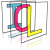Image Component Library (ICL)
icl::core::Line Class Reference

The ICLs abstract line class describing a line from Point "start" to Point "end". More...

`#include <Line.h>`

## Public Member Functions

Line (utils::Point start=utils::Point::null, utils::Point end=utils::Point::null)
Creates a new line from point "start" to point "end". More...

Line (utils::Point start, float angle, float length)
Creates a new line by given polar coordinates. More...

Line operator+ (const utils::Point &p) const
translates a line by a given vector More...

Line operator- (const utils::Point &p) const
translates a line by a given vector (negative direction) More...

float length () const
calculates the euclidean norm of this line More...

utils::Point findClosestPoint (const utils::Point &p) const
returns the point on the line closest to the given point More...

float getMinDist (const utils::Point &p) const
returns the minimum distance of the line to a given point More...

bool intersects (const core::Line &other, utils::Point32f *p=0, float *dstr=0, float *dsts=0) const
return whether the line intersects with the given other line More...

std::vector< utils::Pointsample (const utils::Rect &limits=utils::Rect::null) const
samples this line from start to end point regarding the given limiting rect More...

void swap ()
swaps the lines start and end point internally More...

## Public Attributes

utils::Point start
start point of this line More...

utils::Point end
end point of this line More...

## Static Public Attributes

static const Line null
Null line of length 0 with and and end point 0. More...

## Detailed Description

The ICLs abstract line class describing a line from Point "start" to Point "end".

This Line class provides basic abilities for the description of an abstract line. A line is defined by two Points "start" and "end" where each of this points is a 2D integer vector. Lines can be translated by using the "+"- and "-"-operators. In addition it is possible to sample a line into the discrete grid. Internally this sampling procedure is highly optimized by an implementation of Bresenhams line- algorithm, which draws lines without any floating point operation at all.

## ◆ Line() [1/2]

 icl::core::Line::Line ( utils::Point start = `utils::Point::null`, utils::Point end = `utils::Point::null` )
inline

Creates a new line from point "start" to point "end".

Parameters
 start start point end end point

## ◆ Line() [2/2]

 icl::core::Line::Line ( utils::Point start, float angle, float length )

Creates a new line by given polar coordinates.

Parameters
 start start point of the line angle angle of the line length length of the line

## ◆ findClosestPoint()

 utils::Point icl::core::Line::findClosestPoint ( const utils::Point & p ) const

returns the point on the line closest to the given point

## ◆ getMinDist()

 float icl::core::Line::getMinDist ( const utils::Point & p ) const
inline

returns the minimum distance of the line to a given point

The distance can never be longer then the max-distance to start and end of the line

## ◆ intersects()

 bool icl::core::Line::intersects ( const core::Line & other, utils::Point32f * p = `0`, float * dstr = `0`, float * dsts = `0` ) const

return whether the line intersects with the given other line

Optionally, the actual intersection point can be calculated and stored into a non-null p argument. dstr and dsts are filled with the interpolation factors between start and end for the actual intersection point if not null. If there is no intersection, p, dstr and dsts are not used

## ◆ length()

 float icl::core::Line::length ( ) const

calculates the euclidean norm of this line

Returns
length of the line

## ◆ operator+()

 Line icl::core::Line::operator+ ( const utils::Point & p ) const
inline

translates a line by a given vector

Parameters
 p translation vector
Returns
the translated line

## ◆ operator-()

 Line icl::core::Line::operator- ( const utils::Point & p ) const
inline

translates a line by a given vector (negative direction)

Parameters
 p translation vector
Returns
the translated line

## ◆ sample()

 std::vector icl::core::Line::sample ( const utils::Rect & limits = `utils::Rect::null` ) const

samples this line from start to end point regarding the given limiting rect

Parameters
 limits each line point is check for being inside of this rect the eases working e.g. on image planes, that have an finite extend. If the limits rect has width*height == 0, the limits are not regarded.
Returns
vector of line Points

Please note: for more efficient line sampling, the core::LineSampler class can be used!

## ◆ swap()

 void icl::core::Line::swap ( )
inline

swaps the lines start and end point internally

## ◆ end

 utils::Point icl::core::Line::end

end point of this line

## ◆ null

 const Line icl::core::Line::null
static

Null line of length 0 with and and end point 0.

## ◆ start

 utils::Point icl::core::Line::start

start point of this line

The documentation for this class was generated from the following file:
• /Users/alneuman/vm/icl/ICLCore/src/ICLCore/Line.h